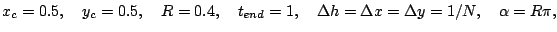Jump:

# Accuracy Study for the Available Cartesian Patch Solvers

We use the exact solution of a stationary rotating vortex for 2D Euler equations as initial conditions and to quantify the error in the density in the L1 norm. This specific exact solution is available under VortexRotationExactSolution. The computational codes are available here: [Clawpack], [WENO], [RIM]

For the following tests we use the computational domain [0,1]x[0.1] and the parameterswhich results in a full rotation of particles positioned at r=R/2. Periodic boundary conditions. For all schemes the measured mass loss is around machine accuracy.

The computational costs for each time step are also measured (test run on a Pentium-4-2.6 GHz CPU).

### One-step methods

• The truly mulit-dimensioanl Wave Propagation Method and dimensional splitting are the schemes inside Clawpack. They are stable up to CFL=1 and for these methods time step adjustment for CFL=0.9 was used.
• Wave Propagation: Method(2)=4, Method(3)=2. Godunov-Splitting: Method(2)=4, Method(3)=-2.
• The Riemann Invariant Manifold (RIM) Method is implemented in the RIM solver. The current implmentation is stable only up to CFL=0.5 and time step adjustment for CFL=0.45 was used.

 N Wave Propagation, Roe solver Godunov-Splitting, Roe solver Godunov-Splitting, Steger-Warming Riemann Invariant Manifold Error Order Cost Error Order Cost Error Order Cost Error Order Cost 20 0.0111235 0.0028 0.0182218 0.0029 0.0165902 0.0028 0.0256279 0.0028 40 0.0037996 1.55 0.0091 0.0090662 1.01 0.0086 0.0119211 0.48 0.0067 0.0139879 0.87 0.0107 80 0.0013388 1.50 0.0426 0.0046392 0.97 0.0434 0.0058922 1.02 0.0353 0.0073075 0.94 0.0465 160 0.0005005 1.42 0.1757 0.0023142 1.00 0.1589 0.0029312 1.01 0.1145 0.0037130 0.97 0.2145

• One step of RIM is slightly more expensive than with Clawpack. Both implementation are in Fortran 77.
• The current implementation of RIM is less accurate and efficient than standard schemes. The implementation is provided for comparisons, but the approach is currently not developed further.

### Three-stage Runge-Kutta methods

• WENO and TCD are the essential two schemes inside the WENO-TCD solver. For both methods a time step adjustment for CFL=0.6 was used.
• WENO-5 Pt: Span=3, Stencil=5, Method=0. WENO-7 Pt: Span=5, Stencil=7, Method=0. TCD with Method=1 instead.

 N WENO - 5 Point WENO - 7 Point TCD - 5 Point Error Order Cost Error Order Cost Error Order Cost 20 0.0184102 0.0228 0.0082823 0.0316 0.0086420 0.0004 40 0.0069655 1.40 0.0875 0.0020886 1.99 0.1430 0.0044230 0.97 0.0200 80 0.0024369 1.52 0.4792 0.0006971 1.58 0.6998 0.0027805 0.67 0.1131 160 1.7320 2.3133 0.0008734 1.67 0.3870

• WENO is a relatively inefficient as it uses a multi-stage Runge-Kutta for the temporal integration.
• Our implementation uses a vector of state twice as long as required for standard 2D Euler equations and is in Fortran 90.
• A calculation with full Wave-Propagation method at doubled resolution is more accurate and still about 3x faster than with WENO 7-Pt.
• The single components in the WENO-TCD solver can be used for valuable comparisons alone, but for efficiency the dynamic transition from WENO to TCD should always be activated. See WenoHome for details.
• Note that the scheme has been developed for LES simulations for 3D Euler equations. For all other problems the Clawpack-based solvers are currently recommended.

-- RalfDeiterding - 23 Feb 2005

You are here: Amroc > VortexRotationAccuracyStudy

to top